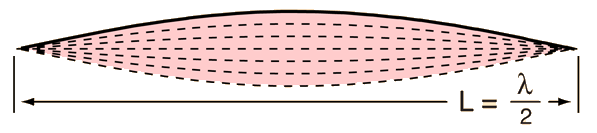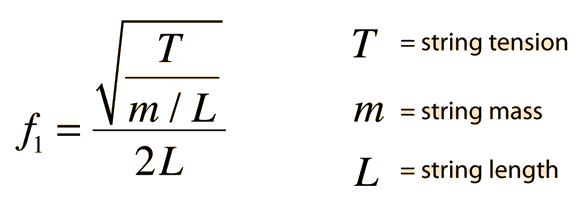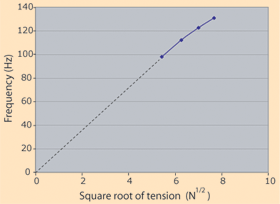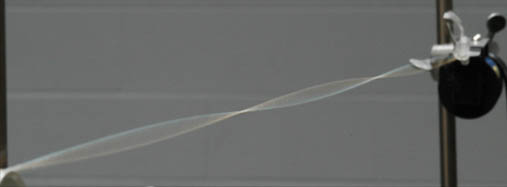# Measurements with a steel string

The fundamental frequency of a stetched string is given by### Calculation

A brief data set with a steel string is plotted to check the consistency of the data with the above equation. Hanging masses provide the tension in the string, which was adjusted to a vibrating length of 50 cm.

 Hanging Mass Tension Frequency 3 kg 29.4 N 98 Hz 4 kg 39.2 N 112 Hz 5 kg 49 N 122.5 Hz 6 kg 58.8 N 131 HzThis is obviously not an ideal string since the best straight line fit of the data of frequency vs square root of tension would not pass through zero frequency at zero tension. If that fact is ignored for the moment and the average slope of the line through zero is taken, the slope is 17.6 Hz/N1/2. Using the frequency relationship above, this corresponds to a mass per unit length m/L = 3.2 grams/meter.This shows a resonant standing wave on a string. It is driven by a vibrator at 120 Hz.

 String frequencies String instruments Illustration with a slinky Mathematical form
Index

Periodic motion concepts

Resonance concepts

 HyperPhysics***** Sound R Nave
Go Back